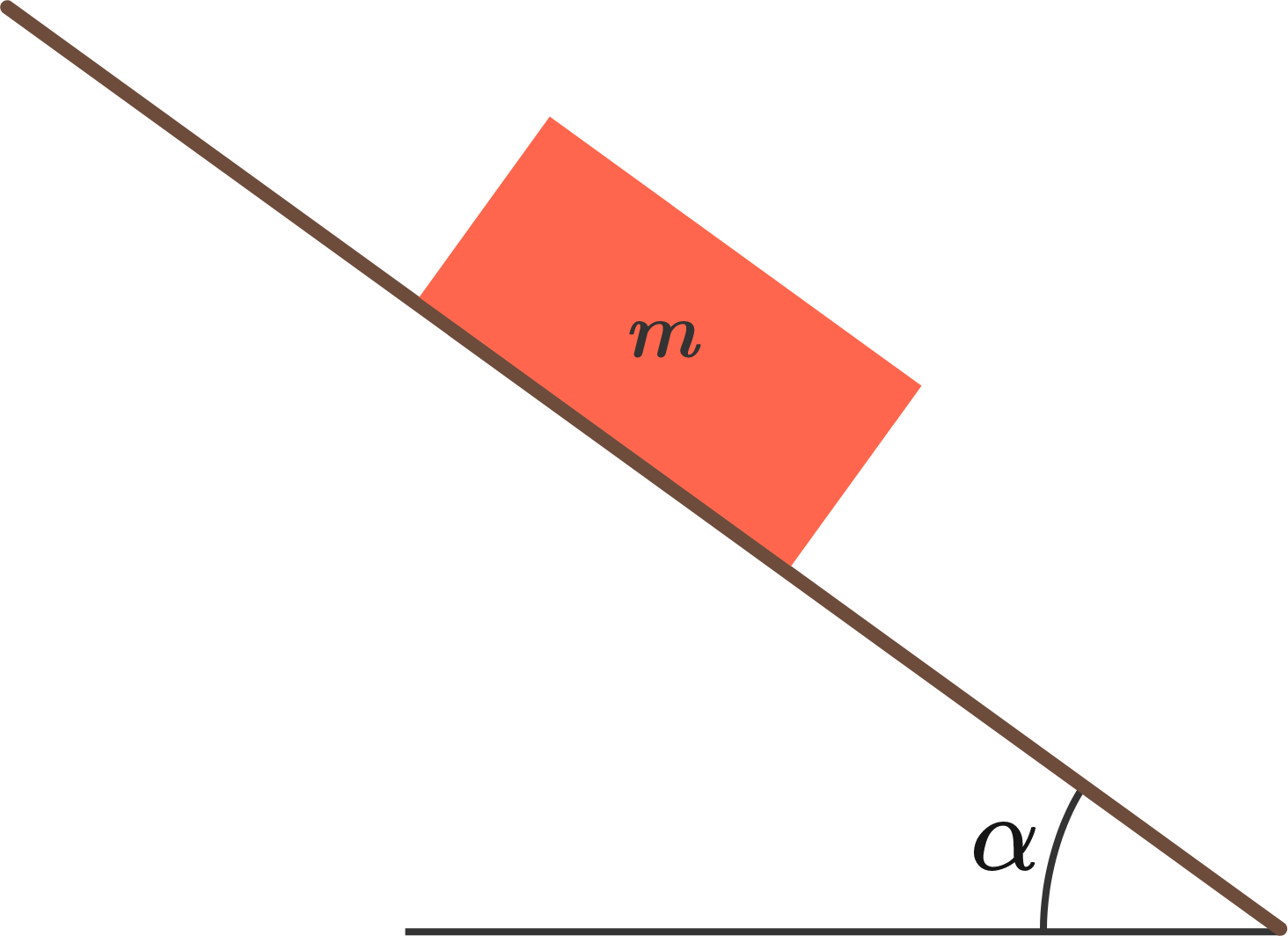# Move a block on an incline by a minimum force

A block of mass $m$ is resting on a rough inclined plane of an angle of inclination $\alpha$. The coefficient of static friction between them is $\mu$. Find the minimum required force to move the block along the incline?


Note: $0<\alpha<\arctan\mu$×